# How to Create a Simple Calculator in Visual Studio 2017

Best way to learn a programming language is building small programs and gradually increasing the complexity of them (At least that’s my opinion). Creating a Simple Calculator is a good point to start learning.

This project is done using Visual Studio Community 2017.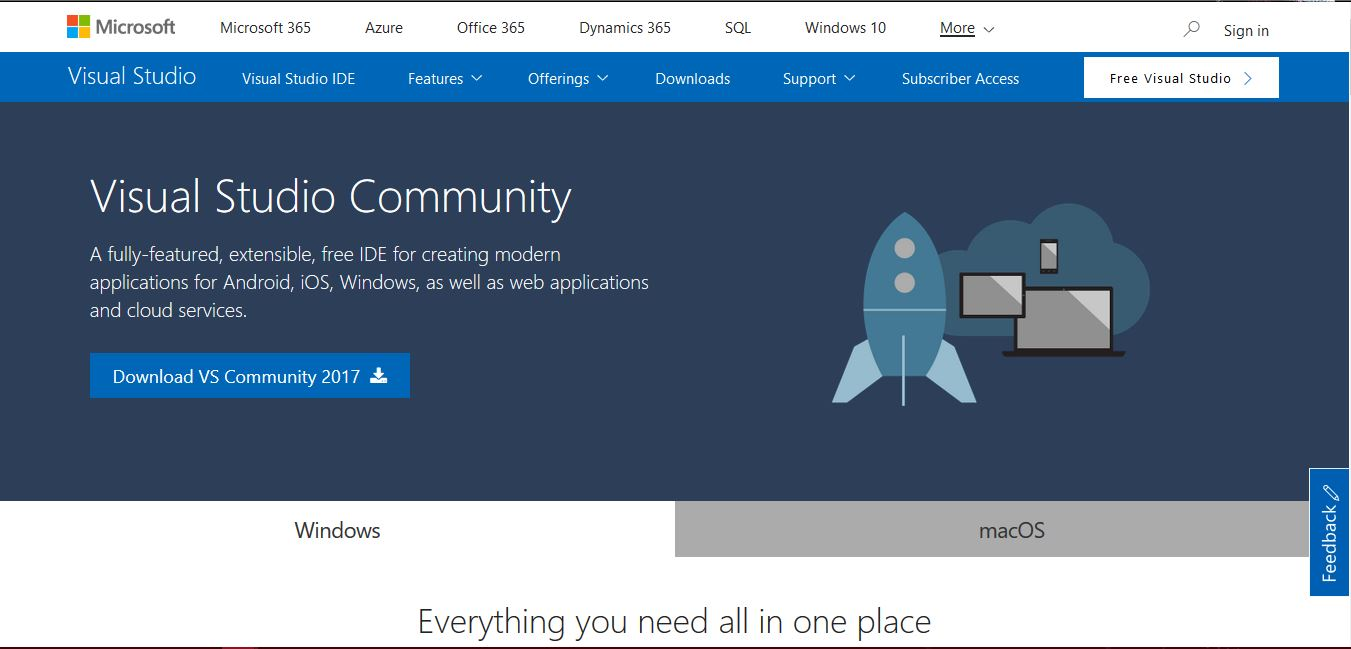You can download and install it from above link. You have to install at least .Net Desktop Development Environment to continue with this project.

After installing and launching the Visual Studio, you have to create a new project.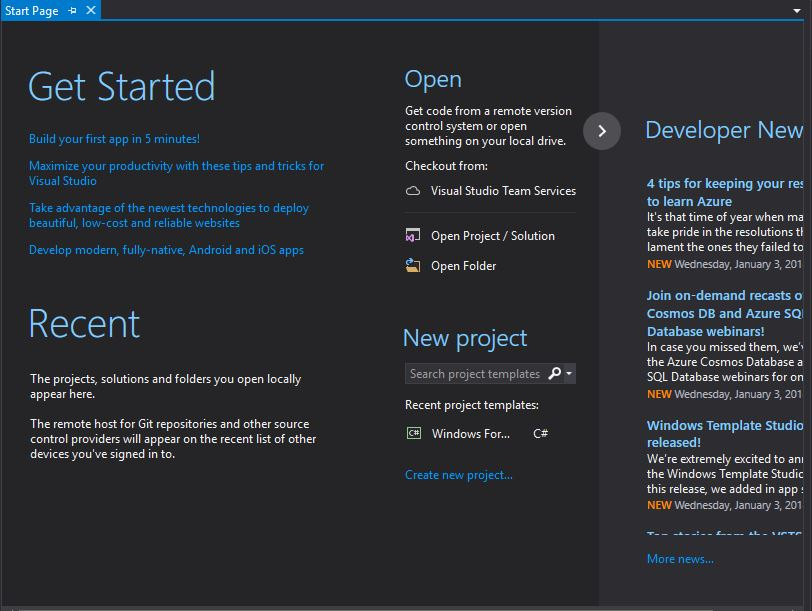Click on create project and select Windows Form App (.NET Framework) under Visual C#. Enter the name of the project, location and click OK to create your project.This will create a project and open the Designer View of the form.Let’s first name the Form. For this,  click and select the form and change the Name Form1 to frmCalc in the property window.You might have notice that at the Top of the form it still shows Form1. We can change it by changing the Text property of the form.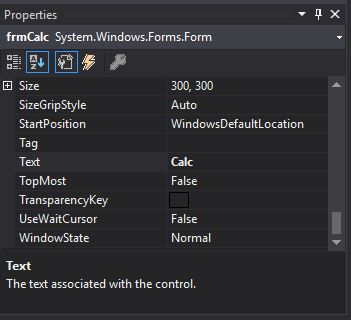Next you have to place;

• 3 TextBoxes
• 2 Labels
• 5 Buttons

as shown in the image below.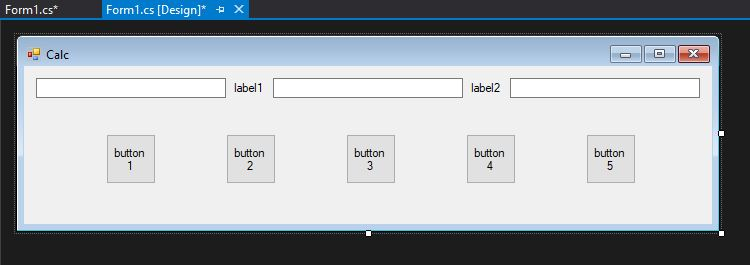You can drag and drop these into Form from the Common Controls in ToolBox.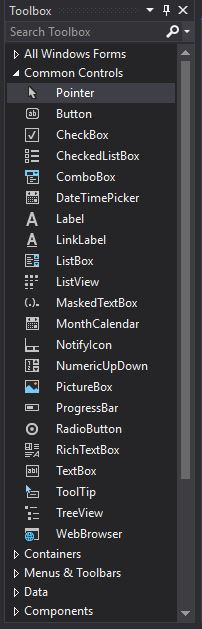(You can open it via View > ToolBox or via Shortcut Ctrl+Alt+X.)

Next change the name and text of Label1 to lblOperation and ? respectively.

Let’s name other controls too.

• TextBox1
• Name – txtNumber1
• TextBox2
• Name – txtNumber2
• TextBox3
• Name – txtAnswer
• Label2
• Name – lblEqual
• Text – =
• Button1
• Name – btnAdd
• Text – +
• Button2
• Name – btnSub
• Text – –
• Button3
• Name – btnDiv
• Text – /
• Button4
• Name – btnMulti
• Text – *
• Button5
• Name – btnClear
• Text – Clear

Now your Form should look like this.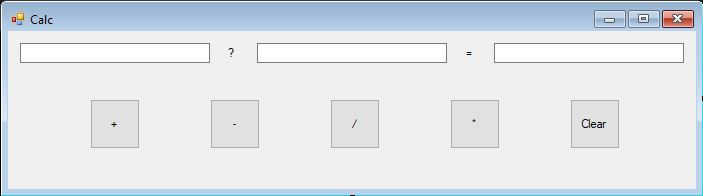As we have created the user interface next thing we have to do is to write some code. Double click on btnAdd to open the code editor.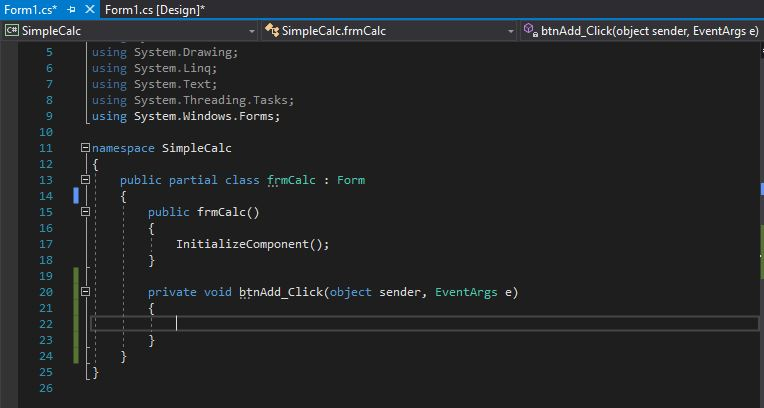First we have to declare 3 variables to store the user entered 2 numbers and the answer.

```double dblNumber1 = 0;
double dblNumber2=0;
double dblAnswer = 0;```

You can add above code inside public partial class frmCalc. It will create 3 variable of double type which have an approximate range of ±5.0 × 10−324 to ±1.7 × 10308

Then add the following code to the btnAdd_Click

```dblNumber1 = Double.Parse(txtNumber1.Text);
dblNumber2 = Double.Parse(txtNumber2.Text);
dblAnswer = dblNumber1 + dblNumber2;
txtAnswer.Text = dblAnswer.ToString();```

This will take the numbers entered into two text boxes, txtNumber1 and txtNumber2, and convert them from string type to double type. Then it will add those two numbers and store in the variable dblAnswer. After that it will change it back into a string and assign as the Text value of txtAnswer text box.

Now as you have written your first bit of code, let’s run this program.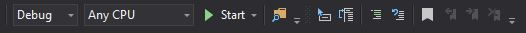You can click the start button or press F5 to run your program (in debug mode).

Now enter two numbers to txtNumber1 and txtNumber2 and click + button. If you have done everything correctly it should show the answer in txtAnswer.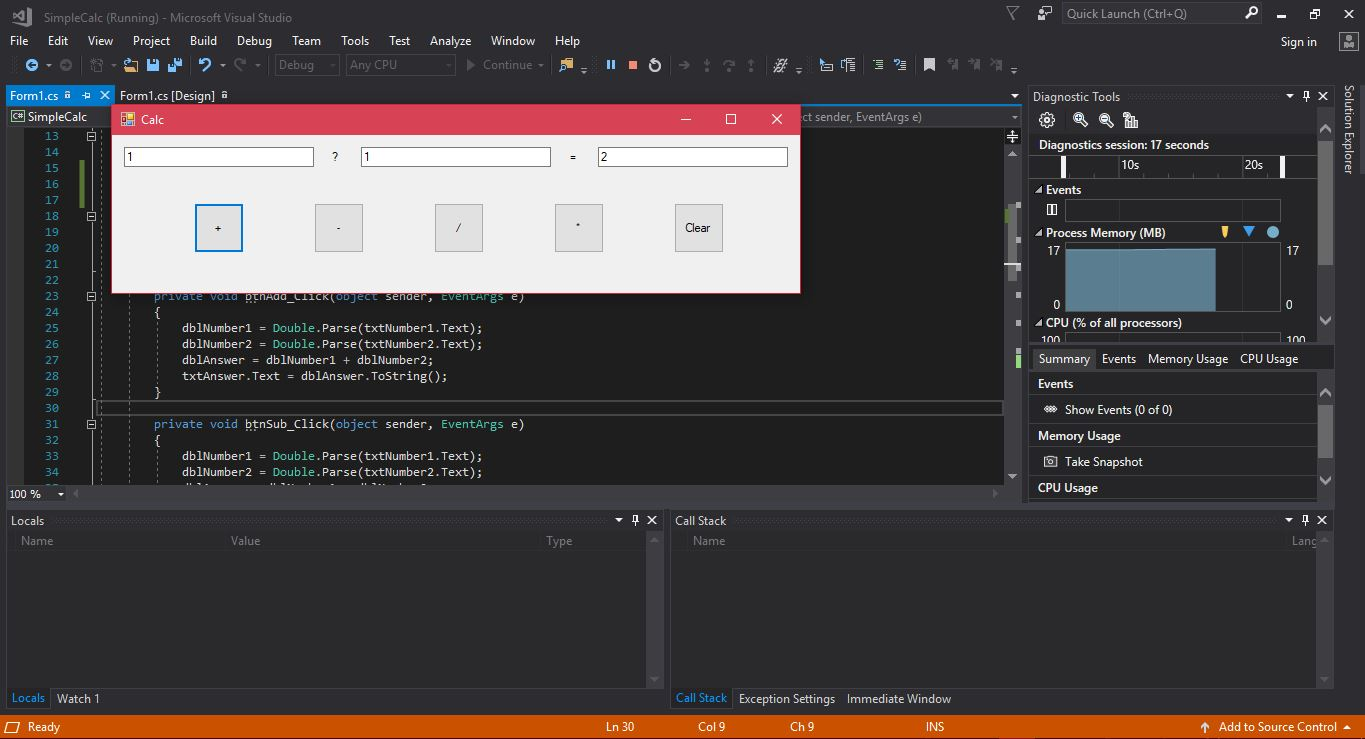Now double click on each button and add the following code at appropriate places. Whole code is included for your convenience.

```using System;
using System.Collections.Generic;
using System.ComponentModel;
using System.Data;
using System.Drawing;
using System.Linq;
using System.Text;
using System.Threading.Tasks;
using System.Windows.Forms;

namespace SimpleCalc
{
public partial class frmCalc : Form
{
double dblNumber1 = 0;
double dblNumber2=0;
double dblAnswer = 0;
public frmCalc()
{
InitializeComponent();
}

private void btnAdd_Click(object sender, EventArgs e)
{
dblNumber1 = Double.Parse(txtNumber1.Text);
dblNumber2 = Double.Parse(txtNumber2.Text);
dblAnswer = dblNumber1 + dblNumber2;
txtAnswer.Text = dblAnswer.ToString();
}

private void btnSub_Click(object sender, EventArgs e)
{
dblNumber1 = Double.Parse(txtNumber1.Text);
dblNumber2 = Double.Parse(txtNumber2.Text);
dblAnswer = dblNumber1 - dblNumber2;
txtAnswer.Text = dblAnswer.ToString();
}

private void btnDiv_Click(object sender, EventArgs e)
{
dblNumber1 = Double.Parse(txtNumber1.Text);
dblNumber2 = Double.Parse(txtNumber2.Text);
dblAnswer = dblNumber1 / dblNumber2;
txtAnswer.Text = dblAnswer.ToString();
}

private void btnMulti_Click(object sender, EventArgs e)
{
dblNumber1 = Double.Parse(txtNumber1.Text);
dblNumber2 = Double.Parse(txtNumber2.Text);
dblAnswer = dblNumber1 * dblNumber2;
txtAnswer.Text = dblAnswer.ToString();
}

private void btnClear_Click(object sender, EventArgs e)
{
double dblNumber1 = 0;
double dblNumber2 = 0;
double dblAnswer = 0;
txtNumber1.Text = "";
txtNumber2.Text = "";
txtAnswer.Text = "";
}
}
}
```

Code for click event of btnSub,btnDiv and btnMulti does the exact same thing as that of btnAdd except they will subtract,divide and multiply the two numbers respectively. Code of the btnClear click event will clear the text of all 3 text boxes and change the values stored in all three variables to zero.

Now you have created a Simple Calculator which can add , subtract , divide and multiply.

This simple calculator has lot of room to improve. For example if you enter some letters instead of a number it will stop working and show an exception. We will improve this calculator in the next article.#### Kavinda

Proud UCSCian | Proud Devan | Computer Geek | Superman | Love Chess & Programming

### 2 thoughts on “How to Create a Simple Calculator in Visual Studio 2017”

This site uses Akismet to reduce spam. Learn how your comment data is processed.